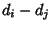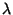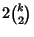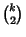## Difference Set

Letbe a Group of Orderandbe a set ofelements of. If the set of differencescontains every Nonzero element ofexactlytimes, thenis a-difference set inof Order. If, the difference set is called planar. The quadratic residues in the Galois Fieldform a difference set. If there is a difference set of sizein a group, thenmust be a multiple of, whereis a Binomial Coefficient.

Gordon, D. M. The Prime Power Conjecture is True for.'' Electronic J. Combinatorics 1, R6 1-7, 1994. http://www.combinatorics.org/Volume_1/volume1.html#R6.